# Deep Learning for Data IntegrationSynergistic effects of data integration with Deep Learning

This is the third article in the series Deep Learning for Life Sciences. In the previous two posts, I showed how to use Deep Learning on Ancient DNA and Deep Learning for Single Cell Biology. Now we are going to discuss how to utilize multiple sources of biological information,** OMICs data**, in order to achieve more accurate modelling of biological systems by Deep Learning.

Biological and biomedical research has been tremendously benefiting last decade from the technological progress delivering DNA sequence (genomics*), gene expression (transcriptomics), protein abundance (proteomics) and many other levels of biological information commonly referred to as OMICs. Despite individual OMICs layers are capable of answering many important biological questions, their combination and consequent synergistic effects from their complementarity promise new insights into behavior of biological systems such as cells, tissues and organisms. Therefore *OMICs integration represents the contemporary challenge in Biology and Biomedicine.

In this article, I will use Deep Learning with Keras and show how integrating multi-OMICs data reveals hidden patterns not visible in individual OMICs.

### Single Cells make Big Data

The problem of data integration is not entirely new for Data Science. Imagine we know that a person looks at certain images, reads certain texts and listens to certain music. Image, text and sound are very different types of data, however we can try to combine those types of data in order to build e.g. a better recommender system which achieves a higher accuracy of capturing the interests of the person. As for Biology and Biomedicine, the idea of data integration has only recently arrived here, however it was actively developed with the biological angle resulting in several interesting methodologies such as mixOmics, MOFA, Similarity Network Fusion (SNF), OnPLS/JIVE/DISCO, Bayesian Networks etc.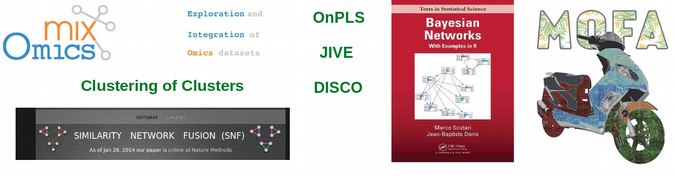Integrative OMICs methods

One problem which all the listed above integrative OMICs methods face is the curse of dimensionality, i.e. inability to work in high-dimensional space with limited number of statistical observations, which is a typical setup for biological data analysis. This is where Single Cell OMICs technologies are very helpful as they deliver hundreds of thousands and even millions of statistical observations (cells) as we discussed in the previous article, and provide thus truly Big Data ideal for integration.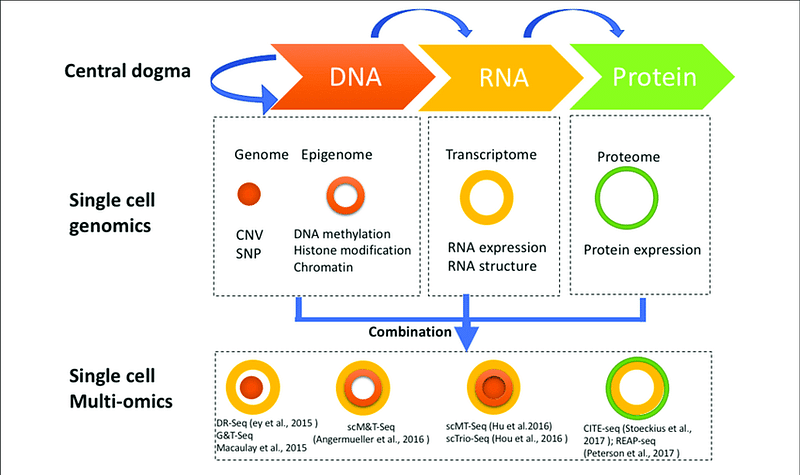Single cell multi-OMICs technologies. Image source

It is very exciting that such multi-OMICs single cell technologies as** [CITEseq](https://www.nature.com/articles/nmeth.4380 "CITEseq")and [scNMTseq](https://www.nature.com/articles/s41467-018-03149-4 "scNMTseq**") provide two and three levels of biological information, respectively, from exactly the same cells.

### Integrating CITEseq data with Deep Learning

Here we will perform unsupervised integration of single cell transcriptomics (scRNAseq) and proteomics (scProteomics) data from CITEseq, 8 617 cord blood mononuclear cells (CBMC), using Autoencoder which is ideally suited for capturing highly non-linear nature of single cell OMICs data. We covered advantages of using Autoencoders for Single Cell Biology in the previous post, but briefly they are related to the fact that single cell analysis is essentially unsupervised. We start by downloading CITEseq data from here, reading them with Pandas and log-transforming, which is equivalent to a mild normalization. As usually, rows are cells, columns are mRNA or protein features, last column corresponds to cell annotation.

``````import numpy as np
import pandas as pd
from keras.models import Model
import matplotlib.pyplot as plt
from sklearn.manifold import TSNE
from keras.utils import plot_model
from keras.layers import Input, Dense
from keras.layers.merge import concatenate

X_scRNAseq = scRNAseq.values[:,0:(scRNAseq.shape-1)]
Y_scRNAseq = scRNAseq.values[:,scRNAseq.shape-1]
X_scProteomics = scProteomics.values[:,0:(scProteomics.shape-1)]
Y_scProteomics = scProteomics.values[:,scProteomics.shape-1]

X_scRNAseq = np.log(X_scRNAseq + 1)
X_scProteomics = np.log(X_scProteomics + 1)
``````

Now we are going to build an Autoencoder model with 4 hidden layers using Keras functional API. The Autoencoder has two inputs, one for each layer of information, i.e. scRNAseq and scProteomics, and corresponding two outputswhich aim to reconstruct the inputs. The two input layers are separately linearly transformed in the first hidden layer (equivalent to PCA dimensionality reduction) before they are concatenated in the second hidden layer. Finally, the merged OMICs are processed through the** bottleneck** of the Autoencoder, and finally the dimensions are gradually reconstructed to the initial ones according to the “butterfly” symmetry typical for Autoencoders.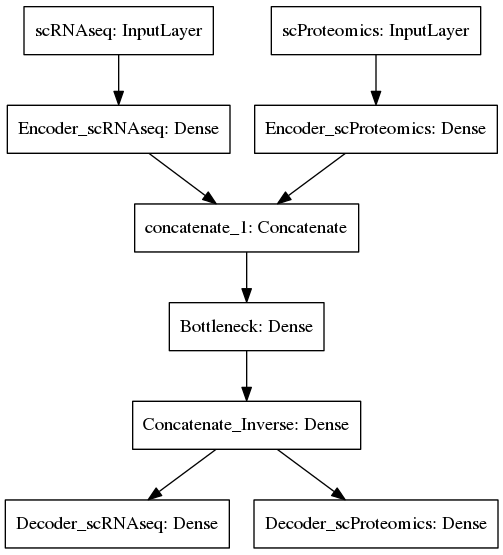Unsupervised integration of CITEseq data

In the code for the Autoencoder below, it is important to note that the first hidden layer imposes severe dimensionality reduction on the scRNAseq from 977 to 50 genes, while it leaves the scProteomics almost untouched, i.e. reduces dimensions from 11 to 10. The bottleneck further reduces the total 60 dimensions after concatenation down to 50 latent variables which represent combinations of both mRNA and protein features.

``````# Input Layer
ncol_scRNAseq = X_scRNAseq.shape
input_dim_scRNAseq = Input(shape = (ncol_scRNAseq, ), name = "scRNAseq")
ncol_scProteomics = X_scProteomics.shape
input_dim_scProteomics = Input(shape = (ncol_scProteomics, ), name = "scProteomics")

# Dimensions of Encoder for each OMIC
encoding_dim_scRNAseq = 50
encoding_dim_scProteomics = 10

# Encoder layer for each OMIC
encoded_scRNAseq = Dense(encoding_dim_scRNAseq, activation = 'linear',
name = "Encoder_scRNAseq")(input_dim_scRNAseq)
encoded_scProteomics = Dense(encoding_dim_scProteomics, activation = 'linear',
name = "Encoder_scProteomics")(input_dim_scProteomics)

# Merging Encoder layers from different OMICs
merge = concatenate([encoded_scRNAseq, encoded_scProteomics])

# Bottleneck compression
bottleneck = Dense(50, kernel_initializer = 'uniform', activation = 'linear',
name = "Bottleneck")(merge)

#Inverse merging
merge_inverse = Dense(encoding_dim_scRNAseq + encoding_dim_scProteomics,
activation = 'elu', name = "Concatenate_Inverse")(bottleneck)

# Decoder layer for each OMIC
decoded_scRNAseq = Dense(ncol_scRNAseq, activation = 'sigmoid',
name = "Decoder_scRNAseq")(merge_inverse)
decoded_scProteomics = Dense(ncol_scProteomics, activation = 'sigmoid',
name = "Decoder_scProteomics")(merge_inverse)

# Combining Encoder and Decoder into an Autoencoder model
autoencoder = Model(input = [input_dim_scRNAseq, input_dim_scProteomics],
output = [decoded_scRNAseq, decoded_scProteomics])

# Compile Autoencoder
loss={'Decoder_scRNAseq': 'mean_squared_error',
'Decoder_scProteomics': 'mean_squared_error'})
autoencoder.summary()
``````

A very handy thing here is that we can assign different loss functions to OMICs coming from different statistical distributions, e.g. combining categorical and continuous data we can apply categorical cross entropy and mean squared error, respectively. Another great thing about data integration via Autoencoders is that all OMICs know about each other as the weights for each node / feature are updated through back propagation in the context of each other. Finally, let us train the Autoencoder and feed the bottleneck into tSNE for visualization:

``````# Autoencoder training
estimator = autoencoder.fit([X_scRNAseq, X_scProteomics],
[X_scRNAseq, X_scProteomics],
epochs = 100, batch_size = 128,
validation_split = 0.2, shuffle = True, verbose = 1)
print("Training Loss: ",estimator.history['loss'][-1])
print("Validation Loss: ",estimator.history['val_loss'][-1])
plt.plot(estimator.history['loss'])
plt.plot(estimator.history['val_loss'])
plt.title('Model Loss')
plt.ylabel('Loss')
plt.xlabel('Epoch')
plt.legend(['Train','Validation'], loc = 'upper right')
plt.show()

# Encoder model
encoder = Model(input = [input_dim_scRNAseq, input_dim_scProteomics],
output = bottleneck)
bottleneck_representation = encoder.predict([X_scRNAseq, X_scProteomics])

# tSNE on Autoencoder bottleneck representation
model_tsne_auto = TSNE(learning_rate = 200, n_components = 2, random_state = 123,
perplexity = 90, n_iter = 1000, verbose = 1)
tsne_auto = model_tsne_auto.fit_transform(bottleneck_representation)
plt.scatter(tsne_auto[:, 0], tsne_auto[:, 1], c = Y_scRNAseq, cmap = 'tab20', s = 10)
plt.title('tSNE on Autoencoder: Data Integration, CITEseq')
plt.xlabel("tSNE1")
plt.ylabel("tSNE2")
plt.show()
``````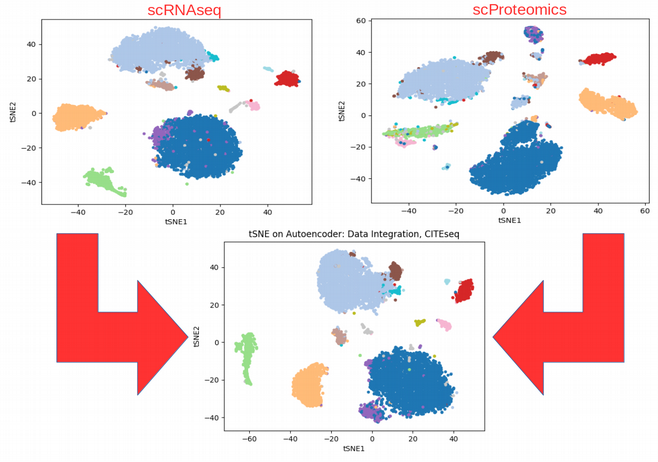Effect of CITEseq data integration: to see patterns invisible in individual OMICs

Comparing the tSNE plots obtained using individual OMICs with the tSNE on the bottleneck of the Autoencoder that combines the data, we can immediately see that the integration somewhat averages and reinforces the individual OMICs. For example, the purple cluster would be hard to discover using the scRNAseq data alone as it is not distinct from the blue cell population, however after integration the purple group of cells is easily distinguishable. This is the power of data integration!

### Integrating scNMTseq data with Deep Learning

While CITEseq includes two single cell levels of information (transcriptomics and proteomics), another fantastic technology, scNMTseq, delivers three OMICs from the same biological cells: 1) transcriptomics (scRNAseq), 2) methylation pattern (scBSseq), and 3) open chromatin regions (scATACseq). The raw data can be downloaded from here.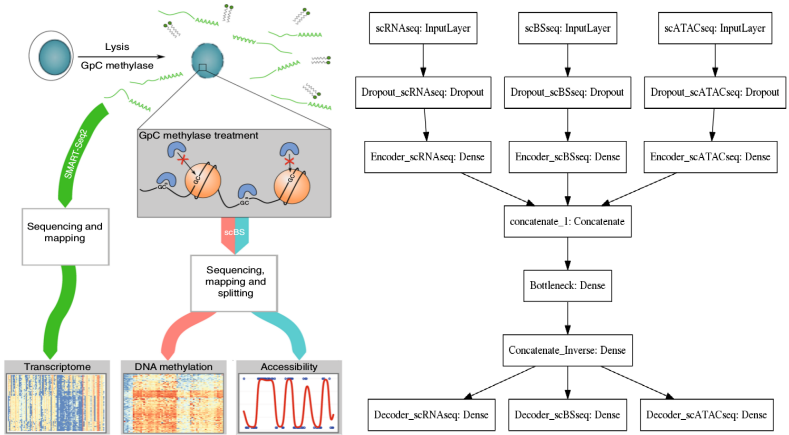scNMTseq data integration with Autoencoder

The architecture of the Autoencoder is analogous to the one used for CITEseq with only one peculiarity: Dropout regularization is used on the input layers. This is due to the fact that we have only ~120 cells sequenced while the dimensionality of the feature space is tens of thousands, so we need to apply regularization to overcome the curse of dimensionality. Note that this was not necessary for CITEseq where we had ~8K cells and ~1K features, so exactly opposite situation. Nevertheless, overall scNMTseq is not an easy case for data integration, I firmly believe though that this is just the beginning of single cell multi-OMICs era and many more cells will arrive soon from this exciting technology, so it is better to be prepared.

``````import numpy as np
import pandas as pd
from umap import UMAP
from keras.models import Model
import matplotlib.pyplot as plt
from keras.layers.merge import concatenate
from keras.layers import Input, Dense, Dropout

################## READ AND TRANSFORM DATA ##################

X_scRNAseq = scRNAseq.values[:,0:(scRNAseq.shape-1)]
Y_scRNAseq = scRNAseq.values[:,scRNAseq.shape-1]
X_scBSseq = scBSseq.values[:,0:(scBSseq.shape-1)]
Y_scBSseq = scBSseq.values[:,scBSseq.shape-1]
X_scATACseq = scATACseq.values[:,0:(scATACseq.shape-1)]
Y_scATACseq = scATACseq.values[:,scATACseq.shape-1]

X_scRNAseq = np.log(X_scRNAseq + 1)
X_scBSseq = np.log(X_scBSseq + 1)
X_scATACseq = np.log(X_scATACseq + 1)

######################## AUTOENCODER ########################
# Input Layer
ncol_scRNAseq = X_scRNAseq.shape
input_dim_scRNAseq = Input(shape = (ncol_scRNAseq, ), name = "scRNAseq")
ncol_scBSseq = X_scBSseq.shape
input_dim_scBSseq = Input(shape = (ncol_scBSseq, ), name = "scBSseq")
ncol_scATACseq = X_scATACseq.shape
input_dim_scATACseq = Input(shape = (ncol_scATACseq, ), name = "scATACseq")

encoding_dim_scRNAseq = 30
encoding_dim_scBSseq = 30
encoding_dim_scATACseq = 30

# Dropout on Input Layer
dropout_scRNAseq = Dropout(0.2, name = "Dropout_scRNAseq")(input_dim_scRNAseq)
dropout_scBSseq = Dropout(0.2, name = "Dropout_scBSseq")(input_dim_scBSseq)
dropout_scATACseq = Dropout(0.2, name = "Dropout_scATACseq")(input_dim_scATACseq)

# Encoder layer for each OMIC
encoded_scRNAseq = Dense(encoding_dim_scRNAseq, activation = 'elu',
name = "Encoder_scRNAseq")(dropout_scRNAseq)
encoded_scBSseq = Dense(encoding_dim_scBSseq, activation = 'elu',
name = "Encoder_scBSseq")(dropout_scBSseq)
encoded_scATACseq = Dense(encoding_dim_scATACseq, activation = 'elu',
name = "Encoder_scATACseq")(dropout_scATACseq)

# Merging Encoder layers from different OMICs
merge = concatenate([encoded_scRNAseq, encoded_scBSseq, encoded_scATACseq])

# Bottleneck compression
bottleneck = Dense(50, kernel_initializer = 'uniform', activation = 'linear',
name = "Bottleneck")(merge)

#Inverse merging
merge_inverse = Dense(encoding_dim_scRNAseq + encoding_dim_scBSseq +
encoding_dim_scATACseq,
activation = 'elu', name = "Concatenate_Inverse")(bottleneck)

# Decoder layer for each OMIC
decoded_scRNAseq = Dense(ncol_scRNAseq, activation = 'sigmoid',
name = "Decoder_scRNAseq")(merge_inverse)
decoded_scBSseq = Dense(ncol_scBSseq, activation = 'sigmoid',
name = "Decoder_scBSseq")(merge_inverse)
decoded_scATACseq = Dense(ncol_scATACseq, activation = 'sigmoid',
name = "Decoder_scATACseq")(merge_inverse)

# Combining Encoder and Decoder into an Autoencoder model
autoencoder = Model(input = [input_dim_scRNAseq, input_dim_scBSseq,
input_dim_scATACseq],
output = [decoded_scRNAseq, decoded_scBSseq, decoded_scATACseq])

# Compile Autoencoder
loss={'Decoder_scRNAseq': 'mean_squared_error',
'Decoder_scBSseq': 'binary_crossentropy',
'Decoder_scATACseq': 'binary_crossentropy'})
autoencoder.summary()

# Autoencoder training
estimator = autoencoder.fit([X_scRNAseq, X_scBSseq, X_scATACseq],
[X_scRNAseq, X_scBSseq, X_scATACseq], epochs = 130,
batch_size = 16, validation_split = 0.2,
shuffle = True, verbose = 1)
print("Training Loss: ",estimator.history['loss'][-1])
print("Validation Loss: ",estimator.history['val_loss'][-1])
plt.plot(estimator.history['loss']); plt.plot(estimator.history['val_loss'])
plt.title('Model Loss'); plt.ylabel('Loss'); plt.xlabel('Epoch')
plt.legend(['Train','Validation'], loc = 'upper right')

# Encoder model
encoder = Model(input = [input_dim_scRNAseq, input_dim_scBSseq,
input_dim_scATACseq], output = bottleneck)
bottleneck_representation = encoder.predict([X_scRNAseq, X_scBSseq, X_scATACseq])

############### UNIFORM MANIFOLD APPROXIMATION AND PROJECTION (UMAP) ###############
model_umap = UMAP(n_neighbors = 11, min_dist = 0.1, n_components = 2)
umap = model_umap.fit_transform(bottleneck_representation)
plt.scatter(umap[:, 0], umap[:, 1], c = Y_scRNAseq, cmap = 'tab10', s = 10)
plt.title('UMAP on Autoencoder: Data Integration, scNMTseq')
plt.xlabel("UMAP1"); plt.ylabel("UMAP2")
``````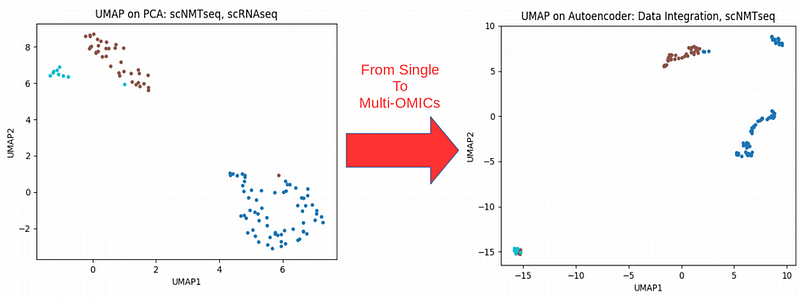Combining transcriptomics with epigenetics information for scNMTseq

Here out of curiosity I fed the bottleneck of the Autoencoder that combines the three scNMTseq OMICs into Uniform Manifold Approximation and Projection (UMAP) non-linear dimensionality reduction technique which seems to outperform tSNE in sense of scalability for large amounts of data. We can immediately see that the homogeneous in sense of gene expression blue cluster splits into two clusters when scRNAseq is combined withepigenetics** information** from the same cells (scBSseq and scATACseq). Therefore it seems that we have captured a new heterogeneity between cells which was hidden when looking only at gene expression scRNAseq data. Can this be a new way of classifying cells across populations by using the whole complexity of their biology? If so, then the question comes: what is a cell population or cell type? I do not know the answer for this question.

### Summary

Here we have learnt that multiple sources of molecular and clinical information are becoming common in Biology and Biomedicine thanks to the recent technological progress. Therefore data integration is a logical next step which provides a more comprehensive understanding of the biological processes by utilizing the whole complexity of the data. Deep Learning framework is ideally suited for data integration due to its truly “integrative” updating of parameters through back propagation when multiple data types learn information from each other. I showed that data integration can result in discoveries of novel patterns in the data which were not previously seen in the individual data types.

As usually, let me know in the comments if you have a specific favorite area in Life Sciences which you would like to address within the Deep Learning framework. Follow me at Medium Nikolay Oskolkov, in twitter @NikolayOskolkov, and check out the codes for this post on my github. I plan to write the next post about Bayesian Deep Learning for patient safety in clinical diagnostics, stay tuned.

## What is Supervised Machine Learning

What is neuron analysis of a machine? Learn machine learning by designing Robotics algorithm. Click here for best machine learning course models with AI

## Pros and Cons of Machine Learning Language

AI, Machine learning, as its title defines, is involved as a process to make the machine operate a task automatically to know more join CETPA

## Artificial Intelligence, Machine Learning, Deep Learning

Artificial Intelligence (AI) will and is currently taking over an important role in our lives — not necessarily through intelligent robots.

## How To Get Started With Machine Learning With The Right Mindset

You got intrigued by the machine learning world and wanted to get started as soon as possible, read all the articles, watched all the videos, but still isn’t sure about where to start, welcome to the club.

## Deep Reinforcement Learning for Video Games Made Easy

Deep Q-Networks have revolutionized the field of Deep Reinforcement Learning, but the technical prerequisites for easy experimentation have barred newcomers until now.